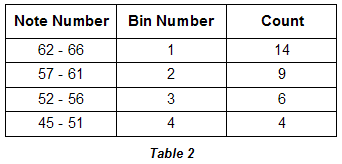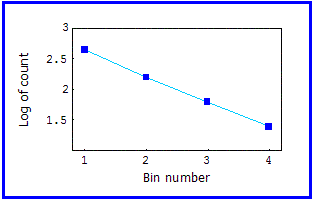Fractal Music

## Second Log-Log Plot

 Under such circumstances, binning the data can reveal patterns. Here is the same data grouped into four roughly equal-sized bins.Here is the log plot with respect to bin number.Using a carefully chosen bin size, the plot illustrates the underlying fact that there approximately 3/2 as many notes in successively higher pitched bins. If, instead of plotting log of the count against respective bin numbers, we choose to make the x-axis the log of the mid-period size of each bin, we would also obtain a straight line. This is because the pitch periods have a logarithmic relationship to each other other through the formula for finding the frequency of a given pitch: Fp = Fref 2d/12 where Fp is the frequency of pitch we wish to calculate, Fref is the frequency of a reference pitch, and d is the intervallic distance between the two pitches as measured in semitones